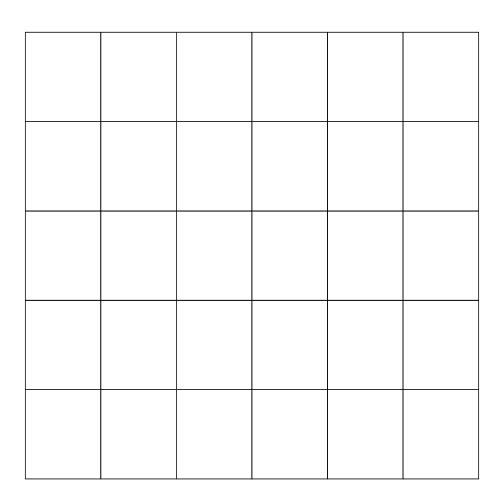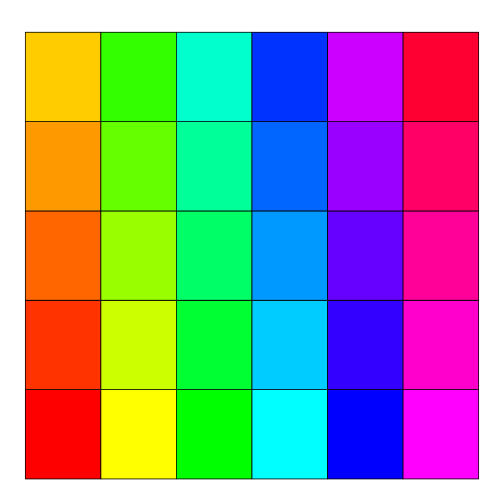# A basic vignette on grids in SOMbrero

## Definition and purpose of a myGrid class object

Objects of class myGrid are made to depict a grid. To be able to create such objects, you have to load the package `SOMbrero`.

## Basic functions on a myGrid class object

In this section, we will consider only the four basic functions that can be applied on a myGrid class object:

• initGrid,
• print.myGrid,
• summary.myGrid,
• plot.myGrid.

### The initGrid function

The initGrid function initializes a new myGrid object. It has 3 parameters:

• `dimension`, which is a vector of two integers. The first one is the x dimension, the second one is the y dimension. The default dimensions are x=5 and y=5,
• `topo`, which is the topology choosen. The value of this argument must be one of these: `square`. Thedefault value is square.
• `dist.type`, which is the distance type. The default value is euclidean.

The following R code initializes a new myGrid object of square topology, x dimension 5 and y dimension 6, and distance type `maximum`.

``````my.first.grid <- initGrid(dimension=c(5,6), topo="square", dist.type="maximum")
``````

### The print.myGrid function

The myGrid object print function prints in the console the main features of the chosen object. The only argument is the object to be printed.

Considering the previously initialized grid, the print command is:

``````print(my.first.grid)
``````
``````##
##       Self-Organizing Map structure
##
##         Features   :
##            topology     :  square
##            x dimension  :  5
##            y dimension  :  6
##            distance type:  maximum
``````

### The summary.myGrid function

The myGrid object summary function is quite simple. It only prints the class of the object and then calls the print function previously described. The only argument is the object to be summarized.

``````summary(my.first.grid)
``````
``````##
## Summary
##
##       Class            :  myGrid
##
##       Self-Organizing Map structure
##
##         Features   :
##            topology     :  square
##            x dimension  :  5
##            y dimension  :  6
##            distance type:  maximum
``````

### The plot.myGrid function

The myGrid object plot function draws the squared area corresponding to the object, in a new graphical window. It has 2 parameters:

• the object to be plotted,
• the color(s) to be used to fill the areas on the graph. The default color is white. If only one color is given, all the neurons will be filled with this color. One can also give many colors: in this case, the number of colors must match the total number of squares. The map is filled from the bottom left corner, up the column and then likewise in the next column to the right.
``````# plot without any color specification: squares are filled in white color
plot(my.first.grid)
````````````# generating colors from the rainbow() function
my.colors <- rainbow(5*6)
plot(my.first.grid, neuron.col=my.colors)
``````